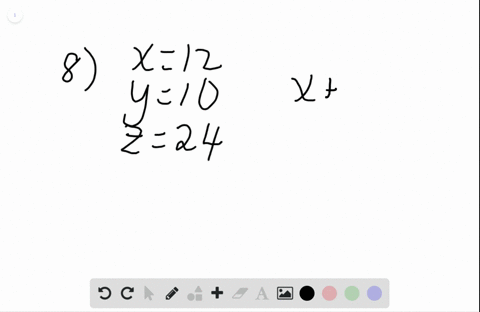Enroll in one of our FREE online STEM bootcamps. Join today and start acing your classes!View Bootcamps### In Exercises $6-14, x=12, y=10,$ and $z=24 .$ Wri…

01:14Problem 7

# In Exercises $6-14, x=12, y=10,$ and $z=24 .$ Write each ratio in simplest form. $=$ to $x$

## Discussion

You must be signed in to discuss.

## Video Transcript

we know we have a ratio of 24 to 12 and this reduces to to toe one because we're dividing these both by 12.